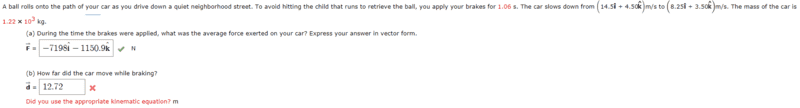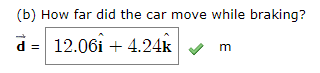# "How far did the car move while braking?"

## Homework Statement

:[/B]

A ball rolls onto the path of your car as you drive down a quiet neighborhood street. To avoid hitting the child that runs to retrieve the ball, you apply your brakes for 1.06 s. The car slows down from 14.5î + 4.50m/s to 8.25î + 3.50m/s.

How far did the car move while braking?

Picture for verification: https://gyazo.com/d3851efe6c4dc5eb7164ef5e72afaf0fNOTE: I already solved the first question (a) in the picture. I'm stuck on (b).

:
[/B]
(vi +vf)/2 = D/t

## The Attempt at a Solution

[([ (14.5i + 8.25k) + (4.50i + 3.50k) ] * 1.06)] / 2 = 12.0575i + 4.24k.
After applying the Pythagorean theorem, my result was 12.78 meters. That answer was rejected.

#### Attachments

Last edited by a moderator:

RPinPA
Homework Helper
I can't see the connection between your "Relevant equations" and your "attempt at a solution". In particular I can't see how all the numbers in your solution are connected to symbols in the equation.

Can you show symbolically what you did with ##(v_i + v_f)/2 = D/t## before putting in the numbers?

kuruman
Homework Helper
Gold Member
2021 Award
You need to find how far the car travels in each direction separately and then combine the displacements to find the distance.

On edit: I got the same distance, 12.78 m, but note that the expected answer is a vector ##\vec d## which you have to enter in unit vector notation.

Last edited:
•Austin Gibson
You need to find how far the car travels in each direction separately and then combine the displacements to find the distance.

[(14.5i + 8.25i) * 1.06] / 2 = 12.0575
[(4.5k + 3.5k) * 1.06] / 2 = 4.24
12.0575 + 4.24 = 16.2975 m <------ ?

kuruman
Homework Helper
Gold Member
2021 Award
Please see the edit in my previous post. You need to enter the displacement as a vector.

•Austin Gibson
RPinPA
Homework Helper
You need to find how far the car travels in each direction separately and then combine the displacements to find the distance.

I wondered about that, but I think it works as a vector equation. Let's see...

$$x_f = x_i + v_{ix} t + 0.5 a_x t^2 \\ y_f = y_i + v_{iy} t + 0.5 a_y t^2$$
becomes
$$\vec D = \vec d_f - \vec d_i \\ = \vec v_i t + 0.5 \vec a t^2 \\ = \vec v_i t + 0.5 \frac {\vec v_f - \vec v_i}{t} t^2 \\ = \vec v_i t + 0.5 \left(\vec v_f - \vec v_i \right) t = 0.5\left(\vec v_f + \vec v_i \right) t$$

So actually you can interpret the displacement equation as a vector equation.

•Austin Gibson
kuruman
Homework Helper
Gold Member
2021 Award
So actually you can interpret the displacement equation as a vector equation.
Yes you can.

•Austin Gibson
Please see the edit in my previous post. You need to enter the displacement as a vector.
Therefore, 16.2975 m in vector form is 12.0575i + 4.24k?

kuruman
Homework Helper
Gold Member
2021 Award
Therefore, 16.2975 m in vector form is 12.0575i + 4.24k?
Yes, enter that and see what happens. And it is not 16.2975 m, it is 12.78 m as entered before (not that it matters).

•Austin Gibson
haruspex
Homework Helper
Gold Member
You need to find how far the car travels in each direction separately and then combine the displacements to find the distance.

On edit: I got the same distance, but note that the expected answer is a vector ##\vec d## which you have to enter in unit vector notation.
Isn't the question flawed? The car has changed direction. If it is skidding the direction will not have changed, or at least not in a predictable way. If it is not skidding then the applied force has changed direction, so we do not know the path taken.
Even if there were no change of direction, we are not told the applied force is constant. If it decelerates very quickly at first and more slowly later it will cover less distance than in the converse case.
Taking the applied force to be constant despite the change of direction would be quite unrealistic.

Yes, enter that and see what happens. And it is not 16.2975 m, it 12.78 m as entered before (not that it matters).Your guidance is appreciated. I calculated a magnitude of 12.78 yesterday (it rejected that), but, as you mentioned, the question demands it in vector notation. If a line is above a variable (in this case "d"), is that to signify vector notation? If that's true, I was oblivious of that until now because the website would prompt me to type the answer in vector form. Therefore, I solved these in the past without recognizing that.

#### Attachments

The first answer was correct (12.0575i + 4.24k), but I was entering the magnitude rather than the answer in vector notation assuming that's what they were requesting.

kuruman
Homework Helper
Gold Member
2021 Award
If a line is above a variable (in this case "d"), is that to signify vector notation?
Yes, it means a vector is expected. Also, it's not a line, it's supposed to be an arrow indicating a vector. However, @haruspex raised a point that I have to think about.

•Austin Gibson
Yes, it means a vector is expected. Also, it's not a line, it's supposed to be an arrow indicating a vector. However, @haruspex raised a point that I have to think about.
The clarification is appreciated.

kuruman